# IBPS RRB 2018 Prelims : Quants – Day 3

Dear Bankersdaily Aspirants,

IBPS RRB PO & Office Assistant 2018 Preliminary Examination starts from 11th August, 2018. The IBPS RRB Exam 2018 is one of the most expected exam of the year. The reason for the consummate expectation is different from that of the others.

The number of candidates attending the IBPS RRB PO & Office Assistant is high when compared to the other exams like SBI & Insurance Exams. The other important factor is that , no English section is there in the preliminary examination of the IBPS RRB PO & Assistant Exams. This is really a boost to the candidates as many of the aspirants couldn’t get the requisite marks in the English section and this exam is the best bet for the aspirants.

Since only few days are left for the IBPS RRB PO & Assistant Exam 2018 Preliminary Exam , we will be providing daily 20 Questions in Quantitative Aptitude & Reasoning Section to evoke the preparation mode.

Aspirants can answer the Questions by commenting the answers for the Questions in the comments section. We will be providing the answers for the Questions @ 6:00 P.M everyday.

## IBPS RRB PO & Assistant 2018 – Prelims Exam – Day 3

Topic : Quantitative Aptitude

Total Number of Questions : 20

Total Time : 15 minutes

Q.1) A alone can complete a piece of work in x days. B is two times as efficient as A. If A and B together can compete the same work in 10 days then, find the number of days taken by B alone to complete the same work?

a) 30 days

b) 20 days

c) 10 days

d) 15 days

e) 40 days

Q.2) Two trains A and B started to move towards each other with the same length. Train A is running at the speed of 90 km/hr and crosses a pole in 20 seconds. If the two trains meet each other in 20 seconds then, find the speed of train B.

a) 75 km/hr

b) 80 km/hr

c) 85 km/hr

d) 90 km/hr

e) 95 km/hr

Q.3) Six years ago, the age of A is 10 years more than B. The sum of the present age of A and B is 50. Find the age of B after 7 years.

a) 37 years

b) 23 years

c) 32 years

d) 17 years

e) 27 years

Q.4) The area of a rectangle is 48 m2. The length and breadth of the rectangle is x+2 and x metres respectively. Find the perimeter of the rectangle.

a) 30 m

b) 32 m

c) 48 m

d) 24 m

e) 28 m

Q.5) Initially A mixture contains milk and water in the ratio of 2:3. If 15 litres of mixture are taken out and it is replaced with water, the ratio becomes 7:18then, how many litres of milk are to be added to get equal quantity of water and milk in the mixture?

a) 26

b) 24

c) 32

d) 22

e) Cannot be determined

Q.6) Five years ago, the population of a city A is 2x lakhs, the male population of the city A is 7 lakhs and the female population of the city A is x lakhs. Find the value of 3x.

a) 14 lakhs

b) 18 lakhs

c) 7 lakhs

d) Cannot be determined

e) 21 lakhs

Q.7) Pipe A alone can fill the tank in 3x minutes. Pipe B alone can fill the tank in 6x minutes. If the two pipes together can fill the tank in 2x minutes, find the value of x.

a) 6 minutes

b) 8 minutes

c) 4 minutes

d) 10 minutes

e) 12 minutes

D.8-12) Find the approximate value of x.

Q.8) 279.9 + 39.8% of 4789.9 + 789.8 = x

a) 2876

b) 2926

c) 2896

d) 2986

e) 2786

Q.9)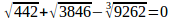a) 54

b) 69

c) 58

d) 62

e) 66

Q.10) 39.89×789.91 – 44.85 ×629.75 = x

a) 3450

b) 3750

c) 3250

d) 4350

e) 3500

Q.11) 7899.75 + 8787.85 + 78.78 + 898.98 – 17.899 = x

a) 17434

b) 17648

c) 16874

d) 17434

e) 18434

Q.12) 64.75% of 2399.75 + 45.01% of 2799.89 = x

a) 2790

b) 2890

c) 2800

d) 2740

e) 2820

D.13-17) Find the missing number from the following series.

Q.13) 250, 500, 1500, 6000, 30000, ?

a) 1800000

b) 120000

c) 210000

d) 150000

e) 180000

Q.14) 15, 8, 9, 15, 32, ?

a) 80

b) 82

c) 82.5

d) 84.5

e) 81.5

Q.15) 2220, 1896, 2257, 1857, 2298, ?

a) 1824

b) 1814

c) 1804

d) 1798

e) 1808

Q.16) 693, 667, 706, 654, 719, ?

a) 636

b) 625

c) 641

d) 671

e) 621

Q.17) 29, 207, 403, 619, 857, ?

a) 1132

b) 1119

c) 1121

d) 1143

e) 1116

D.18-20) Solve the equations and give the relationship between x and y.

Q.18)

I.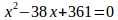II. y2 – 361 = 0

a)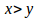b)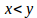c)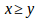d)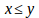e) x=y or the relationship cannot be determined.

Q.19)

I. 2x2 – 7x + 5 =0

II. 4y2 –16x + 16 =0

a)b)c)d)e) x=y or the relationship cannot be determined.

Q.20)

I. 2x2-15x -77 = 0

II. y2– 24y + 143= 0

a)b)c)d)e) x=y or the relationship cannot be determined.

Explanation:

Q.1) (d)

From the question,

Efficiency ratio of A to B = 1: 2

Time ratio of A to B = 2: 1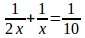X = 15

B alone can complete the work in 15 days

A alone can complete the work in 30 days

Q.2) (d)

From the question,

Length of train A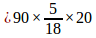= 500 m

Let the speed of train B be x km/hr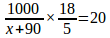X = 90 km/hr

Q.3) (e)

Let the present age of A and B be x and y respectively

X – Y = 10

X + Y = 50

X = 30 years

Y = 20 years

Required age is B = 20 + 7 = 27 years

Q.4) (e)

From the question,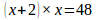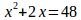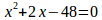X = 6, -8

Therefore, x= 6 m

Required perimeter of the rectangle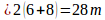Q.5) (d)

From the question,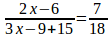X= 10

Now the quantity of milk and water is 14 litres and 36 litres

Hence, 22 litres of milk are needed

Q.6) (e)

From the question,

X lakhs+ 7 lakhs = 2x lakhs

X = 7 lakhs

3x = 21 lakhs

Q.7) (c)

From the question,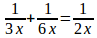X = 4 minutes

Q.8) (d)

280+ 40% of 4790 + 790 = x

2986 =x

Q.9) (d)

21 + 62 – 21= x

62 = x

Q.10) (c)

40× 790 – 45 × 630 = x

31600 – 28350 = x

3250 = x

Q.11) (b)

7900 + 8788 + 79 + 899- 18= x

17648 = x

Q.12) (e)

65% of 2400 + 45% of 2800 = x

1560 + 1260 = x

2820 =x

Q.13) (e)

The series is,

×2, ×3, ×4, ×5, ×6,

Hence, 180000 is the missing number.

Q.14) (c)

The series is,

×0.5+0.5, ×1+1, ×1.5+1.5, ×2+2,×2.5+2.5,

Hence, 82.5 is the missing number

Q.15) (b)

The difference is,

-324, + 361, -400, + 441, -484

Hence, 1814 is the missing number.

Q.16) (c)

The difference is,

-26, +39, -52, +65, -78

Hence, 641 is the missing number.

Q.17) (b)

The difference is,

178, 196, 216, 238, 262

18 20 22 24

Hence, 1119 is the missing number.

Q.18) (c)

From I,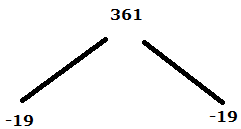Therefore, x = 19, 19

From II,

Y = +19, -19

Hence, x ≥y

Q.19) (e)

From I,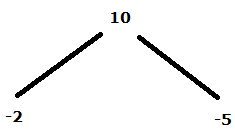Therefore, x = 2/2, 5/2

From II,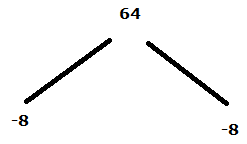Therefore, y = 8/4, 8/4

Hence, Relationship cannot be determined between x and y

Q.20) (d)

From I,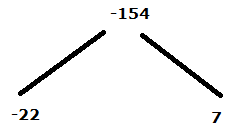Therefore, x = 22/2, -7/2

From II,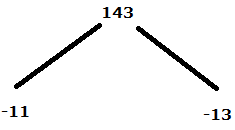Therefore, y= 11, 13

Hence, x≤y

We will post this Practice Questions – Daily till the start of the IBPS RRB PO & Assistant (Regularly)

Quantitative Aptitude @ 3:00 P.M

Reasoning Ability -@ 5:00 P.M

Attend GEEK TEST – SOLVE 10 Daily and enhance your preparation to the next level. Attend the Daily Tests with the Timer and improve your solving ability.

Also Please attend this BLUE WHALE QUESTION (which is a combination of many topics and combined into a single one). This is a One Stop Solution for all your Miscellaneous needs.

## BLUE WHALE CHALLENGE QUESTIONS

Get the FULL LENGTH MOCK TEST SERIES for SBI PO Mains 2018 , SBI CLERK Mains 2018 & BOB PO Mains 2018 from our Bankersdaily Store.